Lecture 8
Within Subjects/Repeated Measures/Paired t

The Concept of Repeated Measures or Within Subjects
As you may be discovering by now, nearly everything in statistics goes by several names. This can be confusing and difficult, but if you understand the underlying concept, it's easier than you might expect.

The concept of repeated measures is pretty easy at first, but then starts to seem more complicated as you go along. Till now, we have compared two groups on a single measure. For instance, comparing two experimental groups on arthritis symptoms. That situation is often called "between subjects" because some subjects in the experiment received the new drug and some received the old drug. Comparison of symptoms was made between different subjects. Experiments are also conducted where the same person receives both drugs at different times. For example, a single patient might start the study taking aspirin, then after two weeks, the new drug is started. Symptoms in the first phase of the study are counted and symptoms in the second phase are counted. This is called repeated measures, because the measure is repeated for each subject.

To analyze this type of study, a special type of statistical test is needed--the within-subjects t-test. "Within" is used because the measure (or measures) being examined is said to be nested within each subject. The term "within subjects" is slightly more general than "repeated measures," because this type of t-test is used in cases other than the repeated measures situation. For instance, one could compare two different measures. A standardized math test might be compared to a standardized verbal test, with the hypothesis that the students in the sample have stronger verbal skills. Another use of the within-subjects t-test is when subjects are linked or paired together in some way. The best example of this is twin studies. Monozygotic twins are compared on alcoholism or some other dimension. In this case, drinking behavior would be analyzed as if there were repeated measurements of the same person. Each twin pair is considered to be the same in some sense. There are several other ways we can link pairs of subjects though, such as married couples, siblings, or participants matched on age or some other dimension. If participants were matched on age, all participants are first ranked according to their age, then pairs of participants of the same (or very close) age are split into two groups, one member or each pair assigned to each group. Each pair is then kept linked together or "yoked."

So, there are several terms that might be used for this type of test: within-subjects t-test, paired t-test, matched pair t-test, or repeated measures t-test. All refer to the same type of test in which pairs of scores are linked together and compared.

Example
Let's look at an example to see how this test is conducted. Generally, we have the same procedures, and the same sampling variability concepts are needed. Let's assume that a local in-home care service makes regular home visits. There are seven nurses and nurses aids in the company that visit patients' homes to provide assistance. The company director decides that a new visit scheduling system should be applied that organizes visits by location to increase the number of visits per day and thus the number of patients that can be served. The company's service region is divided into sectors, and homes located in the same sector are visited by one of the seven health aids on the same day. This table presents the number of visits before and after the new system is implemented.

 ID# for Company's Health Aids Number of Visits/Day Before Number of Visits/Day After 1 3 6 2 8 14 3 4 8 4 6 4 5 9 16 6 2 7 7 12 19

We want to test whether the new scheduling system will significantly increase the number of visits possible. To do this, we need to test whether the increase in visits is simply a chance occurrence or not.

Steps
Generally, we follow the same steps to conduct the significance test and find the confidence intervals as we did with the between-groups test (also called the "between-subjects" t-test), only the formulas are a bit different.

 Step Number Description of Step Specific Example with Between Groups t-test 1. Know the hypothesis you are testing. In this case, we are interested in the average difference between the number of visits before and after the intervention. In the population, that would be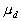. If there is not change, the average difference will be 0, if there is some change it will be larger or smaller than 0.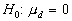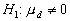2. Use the formula, finding the value of the standard error estimate first. Find the variance of difference scores (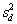), then the standard error of the differences (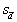). (There are two equivalent formulas forand you can use whichever you prefer.)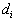stands for the difference between the before and after score for each individual, and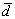stands for the average of all of these differences ().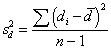, or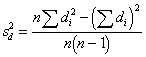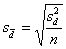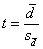3. Check to see if the calculated value indicates significance. To do this: determine the degrees of freedom, and look up the critical value in the table in the back of the book for alpha=.05 (in Daniel's tables, the subscript value .975 is used). If the value you calculated exceeds the value in the table, it is significant (i.e., the null hypothesis is rejected). d.f. = n - 1 4. Calculate the 95% confidence interval. Two values are used: (1) the low value, which subtracts the product of the critical value times the standard error, and (2) the high value, which adds that product.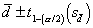Computations

 ID Before After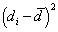1 3 6 3 -1.29 1.66 2 8 14 6 1.71 2.92 3 4 8 4 -.29 .08 4 6 4 -2 6.29 39.56 5 9 16 7 2.71 7.34 6 2 7 5 .71 .50 7 12 19 7 2.71 7.34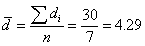,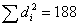,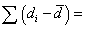59.40

I'll calculate the variance of the difference both ways, but you don't have to.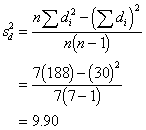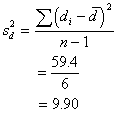Now, the standard error of the differences.And the t value: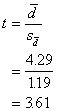To see if this computed value is significant, we should compare it to the tabled value (Table E), looking up under d.f. = n - 1 = 6. The critical value to exceed is t.975 = 2.4496. Because our calculated value of t for the sample, 3.61, is larger than 2.4496 found in the table, we decide our difference is large enough and therefore significant. We reject H0 which stated that there was no difference between before and after measurements.

Interpretation
Note that there is a similarity between this t formula and the between-subjects t formula. Essentially, we are finding a ratio of the average differences between scores relative to sampling variability. So, the average difference () is divided by the standard error of the difference.

The unique thing about this test is that we compare every individual's score to his/her other score, by subtracting the second score from the first (). Then we find the average of that. In the between-groups t-test, we computed the mean of score for one group and then compared it to the mean score of the other group. With the within-subject approach differences are found first, then the average, and with the between-subjects we find the average first, then the difference.

Because each person (or pair) has his or her own control, we will have less overall variation in the sample than if we compare different people in two different groups. And because we can reduce the overall variation, the estimate of sampling variability will be smaller (remember thatis estimated using the variability in the sample,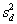). In other words,will generally be smaller than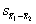.